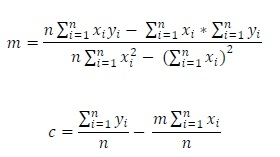# Linear Regression

From a given set of data points, the linear regression finds an equation of straight line. The given points will follow the straight line. Using this formula, we can predict what will be the value for some other specific point, which is not present in the set currently.

For solving linear regression problems using some data points, we have to follow these formulae:Here the m and c are the slope and the y-intercept respectively. Using these expressions, we can get the equation of straight line in this form: 𝑦 = 𝑚𝑥 + 𝑐.

## Input and Output

Input:
The (x, y) coordinates of some points. {(1,3), (2,4), (3,5), (4,6), (5,8)}
Output:
The slope: 1.2 The Intercept: 1.6
The equation: y = 1.2x + 1.6

## Algorithm

linReg(coord)

Input: The given set of coordinate points.

Output: The slope m and y-intercept c.

Begin
for i := 1 to n, do
sumX := sumX + coord[i,0]
sumY := sumY + coord[i,1]
sumXsq := sumXsq + (coord[i,0]*coord[i,0])
sumXY := sumXY + (coord[i,0] * coord[i,1])
done

m := (n * sumXY – (sumX*sumY)) / (n * sumXsq – (sumX * sumX))
c := (sumY / n) – (m * sumX)/n
End

## Example

#include<iostream>
#include<cmath>
#define N 5
using namespace std;

void linReg(int coord[N], float &m, float &c) {
float sx2 = 0, sx = 0, sxy = 0, sy = 0;
for(int i = 0; i<N; i++) {
sx += coord[i];    //sum of x
sy += coord[i];   //sum of y

sx2 += coord[i]*coord[i];      //sum of x^2
sxy += coord[i]*coord[i];     //sum of x*y
}

// finding slope and intercept
m = (N*sxy-(sx*sy))/(N*sx2-(sx*sx));
c = (sy/N)-(m*sx)/N;
}

main() {
// this 2d array holds coordinate points
int point[N] = {{1,3},{2,4},{3,5},{4,6},{5,8}};
float m, c;
linReg(point, m, c);
cout << "The slope: " << m << " The Intercept: " << c << endl;
cout << "The equation: " << "y = "<< m <<"x + "<< c;
}

## Output

The slope: 1.2 The Intercept: 1.6
The equation: y = 1.2x + 1.6